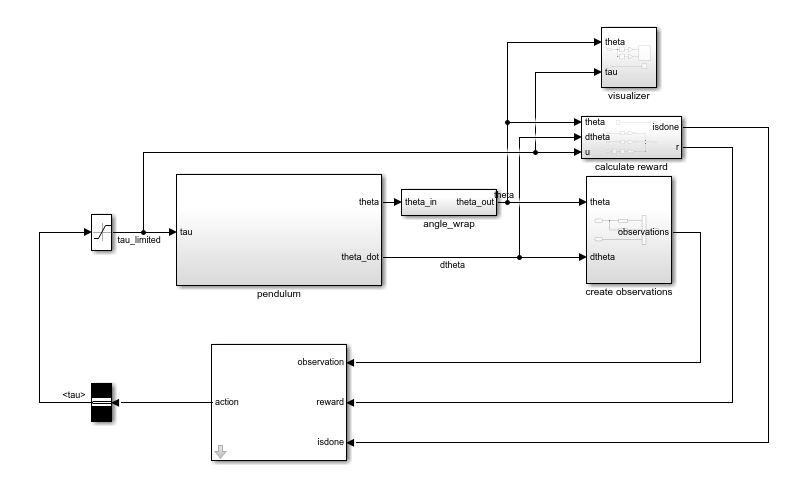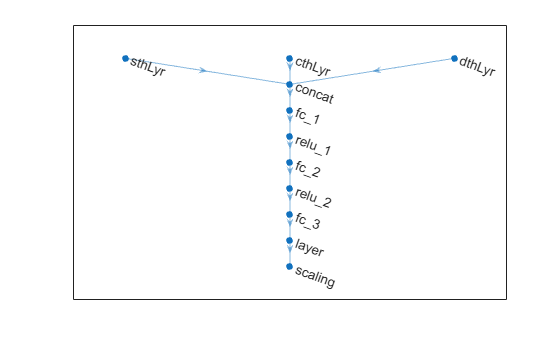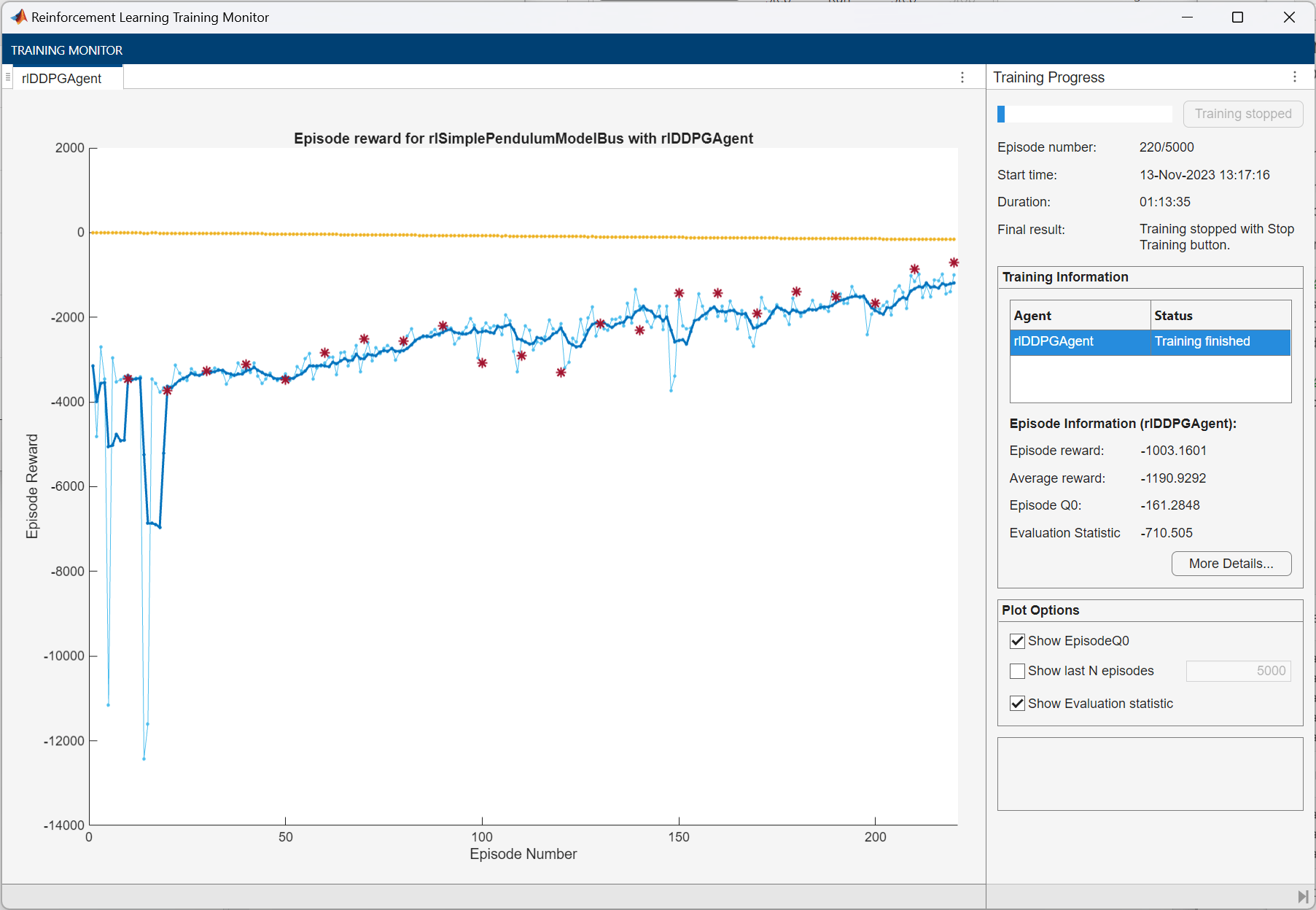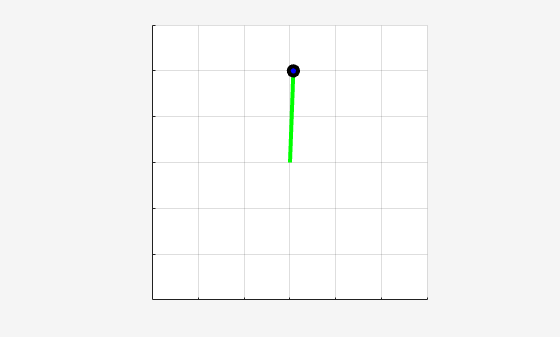Main Content

# Train DDPG Agent to Swing Up and Balance Pendulum with Bus Signal

This example shows how to convert a simple frictionless pendulum Simulink® model to a reinforcement learning environment interface, and trains a deep deterministic policy gradient (DDPG) agent in this environment.

For more information on DDPG agents, see Deep Deterministic Policy Gradient Agents. For an example showing how to train a DDPG agent in MATLAB®, see Train DDPG Agent to Control Double Integrator System.

### Pendulum Swing-Up Model with Bus

The starting model for this example is a simple frictionless pendulum. The training goal is to make the pendulum stand upright without falling over using minimal control effort.

Open the model.

```mdl = 'rlSimplePendulumModelBus'; open_system(mdl)```For this model:

• The upward balanced pendulum position is `0` radians, and the downward hanging position is `pi` radians.

• The torque action signal from the agent to the environment is from –2 to 2 N·m.

• The observations from the environment are the sine of the pendulum angle, the cosine of the pendulum angle, and the pendulum angle derivative.

• Both the observation and action signals are Simulink buses.

• The reward ${\mathit{r}}_{\mathit{t}}$, provided at every time step, is

`${\mathit{r}}_{\mathit{t}}=-\left({{\theta }_{\mathit{t}}}^{2}+0.1{\stackrel{˙}{{\theta }_{\mathit{t}}}}^{2}+0.001{\mathit{u}}_{\mathit{t}-1}^{2}\right)$`

Here:

• ${\theta }_{\mathit{t}}$ is the angle of displacement from the upright position.

• $\stackrel{˙}{{\theta }_{\mathit{t}}}$ is the derivative of the displacement angle.

• ${\mathit{u}}_{\mathit{t}-1}$ is the control effort from the previous time step.

The model used in this example is similar to the simple pendulum model described in Load Predefined Simulink Environments. The difference is that the model in this example uses Simulink buses for the action and observation signals.

### Create Environment Interface with Bus

The environment interface from a Simulink model is created using `rlSimulinkEnv`, which requires the name of the Simulink model, the path to the agent block, and observation and action reinforcement learning data specifications. For models that use bus signals for actions or observations, you can create the corresponding specifications using the `bus2RLSpec` function.

Specify the path to the agent block.

`agentBlk = 'rlSimplePendulumModelBus/RL Agent';`

Create the observation `Bus` object.

```obsBus = Simulink.Bus(); obs(1) = Simulink.BusElement; obs(1).Name = 'sin_theta'; obs(2) = Simulink.BusElement; obs(2).Name = 'cos_theta'; obs(3) = Simulink.BusElement; obs(3).Name = 'dtheta'; obsBus.Elements = obs;```

Create the action `Bus` object.

```actBus = Simulink.Bus(); act(1) = Simulink.BusElement; act(1).Name = 'tau'; act(1).Min = -2; act(1).Max = 2; actBus.Elements = act;```

Create the action and observation specification objects using the Simulink buses.

```obsInfo = bus2RLSpec('obsBus','Model',mdl); actInfo = bus2RLSpec('actBus','Model',mdl);```

Create the reinforcement learning environment for the pendulum model.

`env = rlSimulinkEnv(mdl,agentBlk,obsInfo,actInfo);`

To define the initial condition of the pendulum as hanging downward, specify an environment reset function using an anonymous function handle. This reset function sets the model workspace variable `theta0` to `pi`.

`env.ResetFcn = @(in)setVariable(in,'theta0',pi,'Workspace',mdl);`

Specify the simulation time `Tf` and the agent sample time `Ts` in seconds.

```Ts = 0.05; Tf = 20;```

Fix the random generator seed for reproducibility.

`rng(0)`

### Create DDPG Agent

A DDPG agent decides which action to take, given observations, using an actor representation. To create the actor, first create a deep neural network with three inputs (the observations) and one output (the action). The three observations can be combined using a `concatenationLayer`.

For more information on creating a deep neural network value function representation, see Create Policy and Value Function Representations.

```sinThetaInput = featureInputLayer(1,'Normalization','none','Name','sin_theta'); cosThetaInput = featureInputLayer(1,'Normalization','none','Name','cos_theta'); dThetaInput = featureInputLayer(1,'Normalization','none','Name','dtheta'); commonPath = [ concatenationLayer(1,3,'Name','concat') fullyConnectedLayer(400, 'Name','ActorFC1') reluLayer('Name','ActorRelu1') fullyConnectedLayer(300,'Name','ActorFC2') reluLayer('Name','ActorRelu2') fullyConnectedLayer(1,'Name','ActorFC3') tanhLayer('Name','ActorTanh1') scalingLayer('Name','ActorScaling1','Scale',max(actInfo.UpperLimit))]; actorNetwork = layerGraph(sinThetaInput); actorNetwork = addLayers(actorNetwork,cosThetaInput); actorNetwork = addLayers(actorNetwork,dThetaInput); actorNetwork = addLayers(actorNetwork,commonPath); actorNetwork = connectLayers(actorNetwork,'sin_theta','concat/in1'); actorNetwork = connectLayers(actorNetwork,'cos_theta','concat/in2'); actorNetwork = connectLayers(actorNetwork,'dtheta','concat/in3');```

View the actor network configuration.

```figure plot(actorNetwork)```Specify options for the critic representation using `rlRepresentationOptions`.

`actorOptions = rlRepresentationOptions('LearnRate',1e-4,'GradientThreshold',1);`

Create the actor representation using the specified deep neural network and options. You must also specify the action and observation info for the actor, which you obtained from the environment interface. For more information, see `rlDeterministicActorRepresentation`.

```actor = rlDeterministicActorRepresentation(actorNetwork,obsInfo,actInfo,... 'Observation',{'sin_theta','cos_theta','dtheta'},'Action',{'ActorScaling1'},actorOptions);```

A DDPG agent approximates the long-term reward given observations and actions using a critic value function representation. To create the critic, first create a deep neural network with two inputs, the observation and action, and one output, the state action value.

Construct the critic in a similar manner to the actor. For more information, see `rlQValueRepresentation`.

```statePath = [ concatenationLayer(1,3,'Name','concat') fullyConnectedLayer(400,'Name','CriticStateFC1') reluLayer('Name','CriticRelu1') fullyConnectedLayer(300,'Name','CriticStateFC2')]; actionPath = [ featureInputLayer(1,'Normalization','none','Name', 'action') fullyConnectedLayer(300,'Name','CriticActionFC1','BiasLearnRateFactor', 0)]; commonPath = [ additionLayer(2,'Name','add') reluLayer('Name','CriticCommonRelu') fullyConnectedLayer(1,'Name','CriticOutput')]; criticNetwork = layerGraph(sinThetaInput); criticNetwork = addLayers(criticNetwork,cosThetaInput); criticNetwork = addLayers(criticNetwork,dThetaInput); criticNetwork = addLayers(criticNetwork,actionPath); criticNetwork = addLayers(criticNetwork,statePath); criticNetwork = addLayers(criticNetwork,commonPath); criticNetwork = connectLayers(criticNetwork,'sin_theta','concat/in1'); criticNetwork = connectLayers(criticNetwork,'cos_theta','concat/in2'); criticNetwork = connectLayers(criticNetwork,'dtheta','concat/in3'); criticNetwork = connectLayers(criticNetwork,'CriticStateFC2','add/in1'); criticNetwork = connectLayers(criticNetwork,'CriticActionFC1','add/in2'); criticOpts = rlRepresentationOptions('LearnRate',1e-03,'GradientThreshold',1); critic = rlQValueRepresentation(criticNetwork,obsInfo,actInfo,... 'Observation',{'sin_theta','cos_theta','dtheta'},'Action',{'action'},criticOpts);```

To create the DDPG agent, first specify the DDPG agent options using `rlDDPGAgentOptions`.

```agentOpts = rlDDPGAgentOptions(... 'SampleTime',Ts,... 'TargetSmoothFactor',1e-3,... 'ExperienceBufferLength',1e6,... 'DiscountFactor',0.99,... 'MiniBatchSize',128); agentOpts.NoiseOptions.StandardDeviation = 0.6; agentOpts.NoiseOptions.StandardDeviationDecayRate = 1e-5;```

Then create the DDPG agent using the specified actor representation, critic representation, and agent options. For more information, see `rlDDPGAgent`.

`agent = rlDDPGAgent(actor,critic,agentOpts);`

### Train Agent

To train the agent, first specify the training options. For this example, use the following options:

• Run each training for at most 50000 episodes, with each episode lasting at most `ceil(Tf/Ts)` time steps.

• Display the training progress in the Episode Manager dialog box (set the `Plots` option) and disable the command line display (set the `Verbose` option to `false`).

• Stop training when the agent receives an average cumulative reward greater than –740 over five consecutive episodes. At this point, the agent can quickly balance the pendulum in the upright position using minimal control effort.

• Save a copy of the agent for each episode where the cumulative reward is greater than –740.

For more information, see `rlTrainingOptions`.

```maxepisodes = 5000; maxsteps = ceil(Tf/Ts); trainOpts = rlTrainingOptions(... 'MaxEpisodes',maxepisodes,... 'MaxStepsPerEpisode',maxsteps,... 'ScoreAveragingWindowLength',5,... 'Verbose',false,... 'Plots','training-progress',... 'StopTrainingCriteria','AverageReward',... 'StopTrainingValue',-740);```

Train the agent using the `train` function. Training this agent is a computationally intensive process that takes several hours to complete. To save time while running this example, load a pretrained agent by setting `doTraining` to `false`. To train the agent yourself, set `doTraining` to `true`.

```doTraining = false; if doTraining % Train the agent. trainingStats = train(agent,env,trainOpts); else % Load the pretrained agent for the example. load('SimulinkPendBusDDPG.mat','agent') end```### Simulate DDPG Agent

To validate the performance of the trained agent, simulate it within the pendulum environment. For more information on agent simulation, see `rlSimulationOptions` and `sim`.

```simOptions = rlSimulationOptions('MaxSteps',500); experience = sim(env,agent,simOptions);```Download ebook